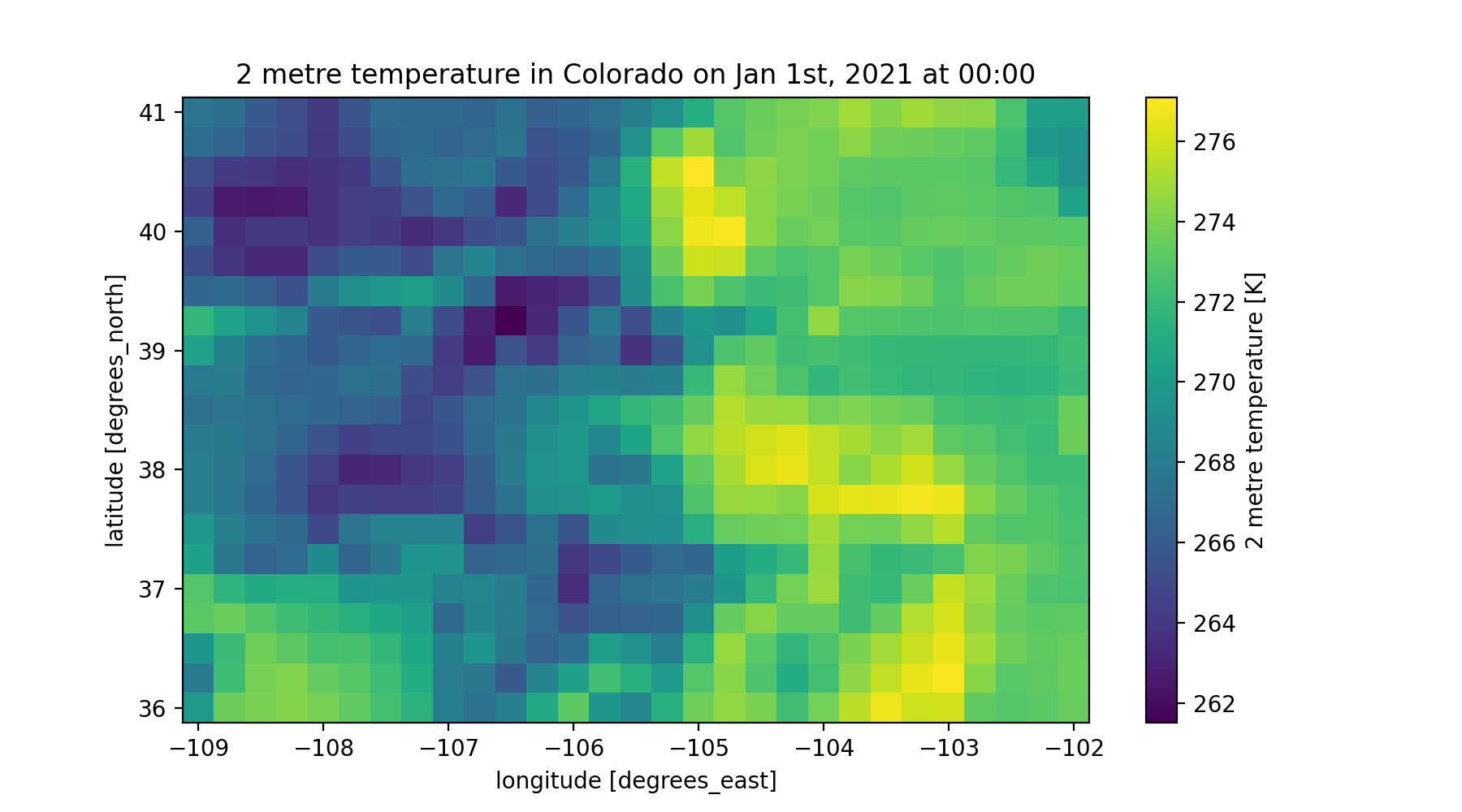pymt_era5 is a package that converts ERA5 datasets into a reusable, plug-and-play data component for PyMT modeling framework developed by Community Surface Dynamics Modeling System (CSDMS). This allows ERA5 datasets (currently support 3 dimensional data) to be easily coupled with other datasets or models that expose a Basic Model Interface.

# Installing pymt¶

Installing pymt from the conda-forge channel can be achieved by adding conda-forge to your channels with:

```conda config --add channels conda-forge
```

Note: Before installing pymt, you may want to create a separate environment into which to install it. This can be done with,

```conda create -n pymt python=3
conda activate pymt
```

Once the conda-forge channel has been enabled, pymt can be installed with:

```conda install pymt
```

It is possible to list all of the versions of pymt available on your platform with:

```conda search pymt --channel conda-forge
```

# Installing pymt_era5¶

Please make sure to first install the CDS API and then follow the command below to install pymt_era5:

```pip install pymt_era5
```

# Coding Example¶

You can learn more details about the coding example from the tutorial notebook.

```import matplotlib.pyplot as plt
import numpy as np

from pymt.models import Era5

# initiate a data component
data_comp = Era5()
data_comp.initialize('config_file.yaml')

# get variable info
for var_name in data_comp.output_var_names:
var_unit = data_comp.var_units(var_name)
var_location = data_comp.var_location(var_name)
var_type = data_comp.var_type(var_name)
var_grid = data_comp.var_grid(var_name)
var_itemsize = data_comp.var_itemsize(var_name)
var_nbytes = data_comp.var_nbytes(var_name)

print('variable_name: {} \nvar_unit: {} \nvar_location: {} \nvar_type: {} \nvar_grid: {} \nvar_itemsize: {}'
'\nvar_nbytes: {} \n'. format(var_name, var_unit, var_location, var_type, var_grid, var_itemsize, var_nbytes))

# get time info
start_time = data_comp.start_time
end_time = data_comp.end_time
time_step = data_comp.time_step
time_units = data_comp.time_units
time_steps = int((end_time - start_time)/time_step) + 1

print('start_time: {} \nend_time: {} \ntime_step: {} \ntime_units: {} \ntime_steps: {}'.format(
start_time, end_time, time_step, time_units, time_steps))

# get variable grid info
grid_type = data_comp.grid_type(var_grid)
grid_rank = data_comp.grid_ndim(var_grid)
grid_shape = data_comp.grid_shape(var_grid)
grid_spacing = data_comp.grid_spacing(var_grid)
grid_origin = data_comp.grid_origin(var_grid)

print('grid_type: {} \ngrid_rank: {} \ngrid_shape: {} \ngrid_spacing: {} \ngrid_origin: {}'.format(
grid_type, grid_rank, grid_shape, grid_spacing, grid_origin))

# get variable data
data = data_comp.get_value('2 metre temperature')
data_2D = data.reshape(grid_shape)
print(data.shape, data_2D.shape)

# get X, Y extent for plot
min_y, min_x = grid_origin
max_y = min_y + grid_spacing*(grid_shape-1)
max_x = min_x + grid_spacing*(grid_shape-1)
dy = grid_spacing/2
dx = grid_spacing/2
extent = [min_x - dx, max_x + dx, min_y - dy, max_y + dy]

# plot data
fig, ax = plt.subplots(1,1, figsize=(9,5))
im = ax.imshow(data_2D, extent=extent)
cbar = fig.colorbar(im)
cbar.set_label('2 metre temperature [K]')
plt.xlabel('longitude [degree_east]')
plt.ylabel('latitude [degree_north]')
plt.title('2 metre temperature in Colorado on Jan 1st, 2021 at 00:00')

# complete the example by finalizing the component
data_comp.finalize()
```